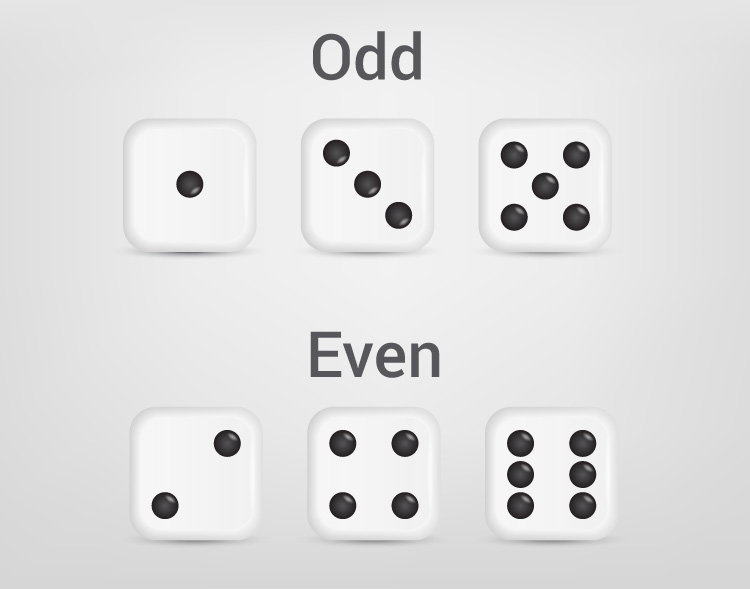# C Program to Check Whether a Number is Even or Odd

In this example, if...else statement is used to check whether a number entered by the user is even or odd.To understand this example, you should have the knowledge of following C programming topics:

An even number is an integer that is exactly divisible by 2. Example: 0, 8, -24

An odd number is an integer that is not exactly divisible by 2. Example: 1, 7, -11, 15

## Example #1: Program to Check Even or Odd

``````#include <stdio.h>
int main()
{
int number;

printf("Enter an integer: ");
scanf("%d", &number);

// True if the number is perfectly divisible by 2
if(number % 2 == 0)
printf("%d is even.", number);
else
printf("%d is odd.", number);

return 0;
}
``````

Output

```Enter an integer: -7
-7 is odd.```

In the program, integer entered by the user is stored in variable number.

Then, whether the number is perfectly divisible by 2 or not is checked using modulus operator.

If the number is perfectly divisible by 2, test expression `number%2 == 0` evaluates to 1 (true) and the number is even.

However, if the test expression evaluates to 0 (false), the number is odd.

## Example #2: Program to Check Odd or Even Using Conditional Operator

``````#include <stdio.h>
int main()
{
int number;

printf("Enter an integer: ");
scanf("%d", &number);

(number % 2 == 0) ? printf("%d is even.", number) : printf("%d is odd.", number);

return 0;
}
``````